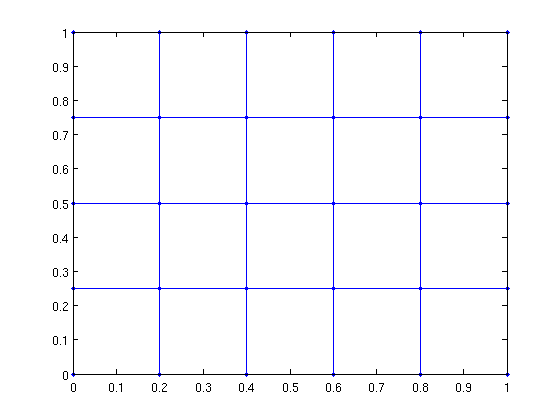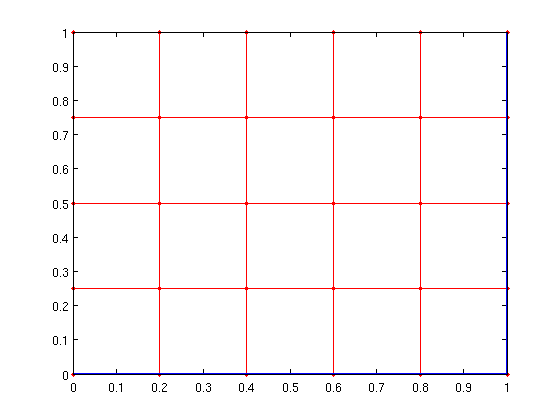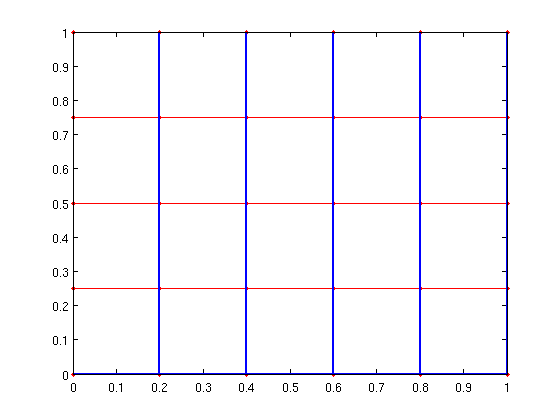# New features in MatlabBGL version 3.0

Although MatlabBGL 3.0 was never officially released, here are some of it's key features.

## Better performance

We redid the backend interface to the BGL routines. This optimization gave a considerable performance increase.

test_benchmark on MatlabBGL 2.1

```2008-10-07, Version 2.1, Matlab 2007b, boost 1.33.0,
g++-3.4 (lib), gcc-? (mex)```
```       airfoil       west    cs-stan    minneso      tapir
large   0.223 s    0.024 s    0.390 s    0.073 s    0.046 s
med     NaN s    0.955 s      NaN s      NaN s    6.621 s
small     NaN s    0.758 s      NaN s      NaN s      NaN s```

test_benchmark on MatlabBGL 3.0

```2008-10-07: Version 3.0, Matlab 2007b, boost 1.34.1,
g++-4.0 (lib), gcc-? (mex)```
```       airfoil       west    cs-stan    minneso      tapir
large   0.183 s    0.017 s    0.222 s    0.048 s    0.037 s
med     NaN s    0.593 s      NaN s      NaN s    3.901 s
small     NaN s    0.543 s      NaN s      NaN s      NaN s```

## Graph construction functions

MatlabBGL 2.1 had a few graph construction functions. MatlabBGL 3.0 adds the grid_graph function for line, grid, cube, and hyper-cube graphs

```[G xy] = grid_graph(6,5); gplot(G,xy,'.-');
```In more dimensions...

```[G xyz] = grid_graph(6,5,3);
G = grid_graph(2,2,2,2);
G = grid_graph([3,3,3,3,3]);
```

## Targeted search

The graph search algorithms now let you specify a target vertex that will stop the search early if possible.

```A = grid_graph(50,50);
tic; d = bfs(A,1,struct()); toc
tic; d = bfs(A,1,struct('target',2)); toc
```
```Elapsed time is 0.001580 seconds.
Elapsed time is 0.000671 seconds.
```

Also implemented for astar_search, shortest_paths, and dfs.

## Edge weights

In Matlab, there is no way to create a sparse matrix with a structural non-zero (used for MatlabBGL edges) and a value of 0 (used for MatlabBGL weights). Consequently, it's impossible to run algorithms on graphs where the edge weights are 0.

Consequently, some algorithms now take an 'edge_weight' parameter that allows you to provide a different set of edge weights which allow structural non-zeros and 0 values.

This behavior is a bit complicated, so see the REWEIGHTED_GRAPHS example for more information.

## Matching algorithms

While maximum cardinality bipartite matching is just a call to max-flow, general graph matching algorithms are not. MatlabBGL 3.0 contains the matching algorithms in Boost 1.34.0.

```load('../graphs/matching_example.mat');
m = matching(A);
sum(m>0)/2 % matching cardinality should be 8
```
```ans =

8

```

## New graph statistics

We added a few new statistics functions.

Test for a topological ordering of a graph (only applies to DAGs or directed acyclic graphs)

```n = 10; A = sparse(1:n-1, 2:n, 1, n, n); % construct a simple dag
p = topological_order(A);

test_dag(A)
test_dag(cycle_graph(6)) % a cycle is not acyclic!
```
```ans =

1

ans =

0

```

Core numbers can help identify important regions in a graph. MatlabBGL includes weighted and directed core numbers. Also, the algorithms return the removal time of a particular vertex, which gives interesting graph orderings.

```% See EXAMPLES/CORE_NUMBERS_EXAMPLE
```

New algorithms for clustering_coefficients on weighted and directed graphs.

```A = clique_graph(6) - cycle_graph(6); % A is a clique - a directed cycle
ccfs = clustering_coefficients(A)
B = sprand(A);
ccfs = clustering_coefficients(B)
C = A|A'; % now it's a full clique again
ccfs = clustering_coefficients(C)
```
```ccfs =

0.7600
0.7600
0.7600
0.7600
0.7600
0.7600

ccfs =

0.3480
0.4104
0.4025
0.4191
0.4156
0.4380

ccfs =

1
1
1
1
1
1

```

## Max-flow algorithms

Since Boost added the Kolmogorov max-flow function, we added the full collection of flow algorithms to MatlabBGL.

```load('../graphs/max_flow_example.mat');

push_relabel_max_flow(A,1,8)
kolmogorov_max_flow(A,1,8)
edmunds_karp_max_flow(A,1,8)

max_flow(A,1,8,struct('algname','push_relabel'));
max_flow(A,1,8,struct('algname','kolmogorov'));
max_flow(A,1,8,struct('algname','edmunds_karp'));
```
```ans =

4

ans =

4

ans =

4

```

## Dominator tree

Dominator trees are relations about presidence in certain types of graphs. These are also called flow-graphs.

```load('../graphs/dominator_tree_example.mat');
p = lengauer_tarjan_dominator_tree(A,1);
```

## New utility functions

MatlabBGL 3.0 introduces some new utility functions.

The output of a shortest path algorithm is a predecessor matrix. To convert these predecessor relationships to a path, use the path_from_pred function.

```[A xy] = grid_graph(6,5); n= size(A,1);
[d dt pred] = bfs(A,1); %
path = path_from_pred(pred,n) % sequence of vertices to upper corner
```
```path =

1     2     3     4     5     6    12    18    24    30

```

Let's draw the path

```gplot(A,xy,'r.-');
[px,py]=gplot(sparse(path(1:end-1),path(2:end),1,n,n),xy,'-');
hold on; plot(px,py,'-','LineWidth',2); hold off;
```We can also create a full shortest path tree using the tree_from_pred function.

```T = tree_from_pred(pred);
gplot(A,xy,'r.-');
[px,py]=gplot(T,xy,'-');
hold on; plot(px,py,'-','LineWidth',2); hold off;
```Finally, there are a few new routines to make working with reweighted graphs easier. See EXAMPLES/REWEIGHTED_GRAPHS for information about the INDEXED_SPARSE and EDGE_WEIGHT_INDEX functions.